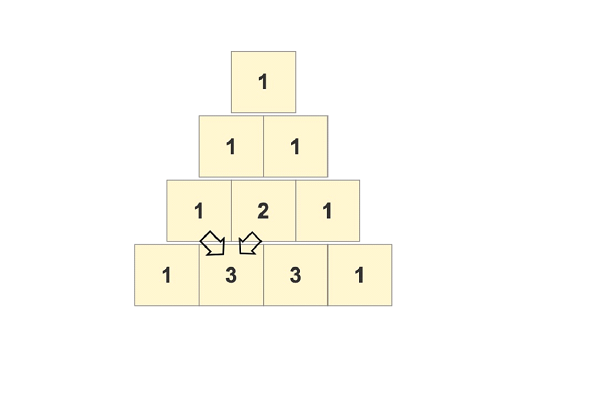# Swift program to print pascal’s triangle

This tutorial will discuss how to write swift program to print Pascal’s triangle.

A pascal’s triangle is a triangular array of the binary coefficient. It is a never ending equilateral triangle which always follows the rule of adding two numbers from the above row to get the number of below row. For example, the initial number of first row is 1 so the number of 2nd row is the sum of two numbers of the 1st row that are 0+1 and 1+0, so we get [1, 1]. Now the number of 3rd rows is the sum of the two numbers of the 2nd row that are 0+1, 1+1, 1+0, so we get 3rd row [1, 2, 1] and so on…

Below is a demonstration of the sameInput

Suppose our given input is −

Total number of rows:
10


Output

The desired output would be −

                          1

1 1

1 2 1

1 3 3 1

1 4 6 4 1

1 5 10 10 5 1

1 6 15 20 15 6 1

1 7 21 35 35 21 7 1

1 8 28 56 70 56 28 8 1

1 9 36 84 126 126 84 36 9 1


## Algorithm

Following is the algorithm −

Step 1 − Create a function

Step 2 − Declare a variable named res to store result.

Step 3 − Check if the row are equal to 0. If yes, then return.

Step 4 − Run a for loop to handle the total number of rows. This loop starts from 0 to row-1(or 0..<row)

Step 5 − Declare another variable named Cres to store the current result.

Step 6 − Run nested for loop to print spaces. This loop starts from 0..<(row-x-1).

Step 7 − Run another nested for loop to print the values of pascal triangle. Here we add two numbers of the above row to find the number of below row.

Step 8 − Calling the function and pass the total number of rows as a parameter.

Step 9 − Print the output.

## Example 1

The following program shows how to to print Pascal’s triangle

import Swift
import Foundation

func createPascalTriangle(row: Int)
{
var res = [[Int]]()
if (row == 0)
{
return
}
for x in 0..<row
{
var Cres = [Int]()
for _ in 0..<(row-x-1)
{
print(" ", terminator:"")
}
for y in 0...x
{
if(x>1 && y>0 && y<x)
{
let val = res[x-1][y] + res[x-1][y-1]
Cres.append(val)
print("\(val) ", terminator: "")
} else {
Cres.append(1)
print("\(1) ", terminator: "")
}
}
res.append(Cres)
print("")
}
}
var num = 5
createPascalTriangle(row:num)



## Output

    1

1 1

1 2 1

1 3 3 1

1 4 6 4 1


Here in the above code, we display the values of pascals triangle in the triangular pattern. Here to find the values of pascals triangle we add two numbers from the above row to get the number of below row using the following code −

for y in 0...x{
if(x>1 && y>0 && y<x){
let val = res[x-1][y] + res[x-1][y-1]
Cres.append(val)
print("\(val) ", terminator: "")
} else {
Cres.append(1)
print("\(1) ", terminator: "")
}


## Example 2

The following program shows how to to print Pascal’s triangle in 1 row

import Swift
import Foundation
func createPascalTriangle(row: Int)->[[Int]]{
var res = [[Int]]()
if (row == 0){
return res
}
for x in 0..<row{
var Cres = [Int]()
for y in 0...x{
if(x>1 && y>0 && y<x){
let val = res[x-1][y] + res[x-1][y-1]
Cres.append(val)
} else {
Cres.append(1)
}
}
res.append(Cres)
}
return res
}
var num = 5
print(createPascalTriangle(row:num))


## Output

[, [1, 1], [1, 2, 1], [1, 3, 3, 1], [1, 4, 6, 4, 1]]


Here in the above code, we display the values of pascals triangle in the array.

Updated on: 13-Dec-2022

213 Views